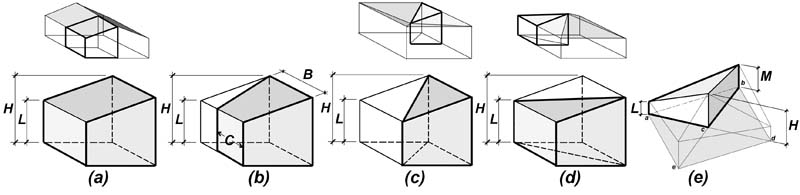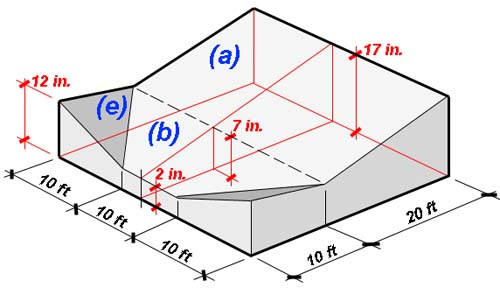Building technology calculators
contact

# Heat loss through roof with tapered insulation calculator

Jonathan Ochshorn

Introduction: The R-value of tapered roof insulation is often found by using the average thickness of the insulation to determine an "average" R-value. However, the true average R-value differs from this assumed value, sometimes significantly. To determine the actual R-value of tapered insulation, the assumed R-value (based on the arithmetic mean average thickness) must be multiplied by the "efficiency" of the insulation, as is done in this calculator.

To look only at the efficiency of tapered insulation, use the tapered insulation efficiency calculator.

A detailed explanation of tapered insulation and R-Values can be found in my paper: Determining the Average R-Value of Tapered Insulation

Directions:

Enter values in all the yellow fields: For each geometric component of the roof (e.g., 1-way tapers, 4-way tapers, crickets, etc.), enter values for high- and low-point thickness (as well as intermediate thickness for crickets only), and ratio of sides B : C for trapezoidal solids only (see Figure 1-b). These various parameters and geometries are illustrated in Figure 1. One cannot separate the R-value calculation of tapered insulation from the R-value calculation of other roof elements such as ceiling finishes or structural slabs: the entire assembly must be evaluated as a unit. For that reason, it is important to include the assumed-constant R-value of all additional roof materials, and the R-value per unit thickness for the tapered insulation.

This calculator assumes typical tapered insulation slopes and does not include the effect of heat-flow trajectories. For steeply sloped tapers, find the efficiency using the tapered insulation efficiency calculator.

Enter roof areas for each component type, and the number of such components. If a component type is not used, enter 0 for "Number of similar components" (row "q," with the green background color).

Press "update" button.Fig. 1. For purposes of calculation, the basic tapered insulation forms are as follows: (a) 1-way slope or 2-way slope; (b) trapezoidal slope with parallel high- and low-point sides; (c) 1-way slope converging down to a point, or 4-way slope with interior drainage; (d) 1-way slope converging up to a point, or 4-way slope with exterior drainage; and (e) triangular cricket

The total "true" heat loss is calculated in row "u." Optionally, by entering values for high- and low-point insulation thickness in row "v," the typically-assumed (and incorrect) heat loss based on the average thickness of the insulation is calculated (row "w").Fig. 2. The default calculations shown below are based on the tapered roof components and dimensions shown here: (a) is a 1-way slope; (b) is a trapezoidal solid; and (e) is a triangular cricket. Tapered insulation types (c) and (d) are not used.

The default calculations shown below are based on the tapered roof components and dimensions shown in Figure 2. It can be seen that the slope of the tapered insulation in this example is 1/2 in. per foot (1:24).